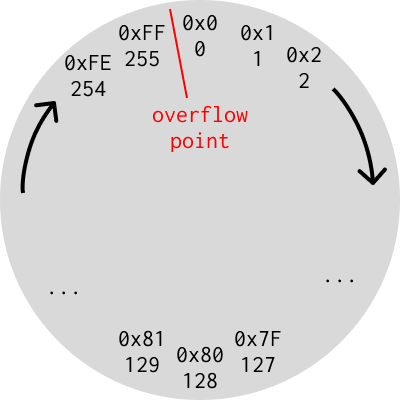## Finally getting two's complement

June 23, 2023

Until recently I understood two's complement as something like: "the way computers represent negative numbers, chosen to make the math work out". If you look at that Wikipedia page there are some recipes, formulas, and mathematical interpretations but I didn't have the intuition for why it works. But now I do, so I'd like to share it with you!

## Unsigned math

First, here's a picture representing a perspective on how ordinary unsigned integers work.Pictured here are the values held in an unsigned byte laid out on a circle. To count upwards you go around the circle clockwise, and downwards you go in reverse. The circle wraps around at 0xFF, the maximum value a byte can hold, back to 0. I marked that point the "overflow point" because if you cross it, the math breaks — taking 3 steps clockwise on this circle from 254 produces 1, not 254+3 = 257.

It's important to keep distinct the ideas of what the actual bits in the computer are (which I write in `0xhex`) versus what number those bits represent (which I write in decimal). For unsigned integers these aren't too interesting because they correspond — e.g. the bit pattern 0x1 represents the integer 1.

## Signed math

Next, here is that same picture, but interpreting the same hex bit patterns as two's complement signed integers — the bit patterns from 0x80 up to 0xFF now represent negative numbers.What's neat about this layout is that the same rotation rule works, even across the negative/positive boundary. Repeating the above example, if you start at -2 (0xFE) and add 3 (take three steps to the right), you end up at 1 (0x1), which is what you'd expect because -2 + 3 = 1. What that means is that, given an arbitrary number in memory, you can actually perform addition and subtraction on it the same without even knowing whether it's representing a signed or unsigned number. Suppose you have the bit pattern 0xFE and you add 0x1 to it, producing 0xFF. If you interpret that operation as unsigned math it's computing 254 + 1 = 255, while if you interpret it as signed math it was -2 + 1 = -1.

Another nice property of this representation is that you can immediately tell whether a number is negative because they all have the highest bit set.

One last nice property of this representation is that positive numbers still look the same as they do in unsigned — 0x1 is still +1.

## Remembering it

How do you compute the bit pattern for a negative signed integer? The formula is simple — `bitwise_not(abs(x)) + 1` — but without an intuition for it, it was hard for me to remember if you add one or subtract one depending on whether you're going from signed to unsigned or the other direction.

Here's an easy trick to remember: you need negative 0 to equal zero. If you bitwise flip 0 you get 0xFF, so you need to add one to produce 0 again. (This paragraph replaced a more complex one based on a helpful comment from `stellalo` on HN!)

PS: I gained the intuition for the above pictures from someone else's website on this topic. I don't remember the site, but I figure repeating it in my own words will also help me remember the lesson better.